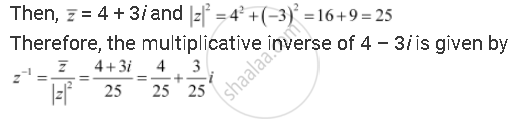CBSE (Arts) Class 11CBSE
Share

# Find the Multiplicative Inverse of the Complex Number 4 – 3i - CBSE (Arts) Class 11 - Mathematics

ConceptAlgebra of Complex Numbers

#### Question

Find the multiplicative inverse of the complex number 4 – 3i

#### Solution

Let z = 4 – 3iIs there an error in this question or solution?

#### Video TutorialsVIEW ALL 

Solution Find the Multiplicative Inverse of the Complex Number 4 – 3i Concept: Algebra of Complex Numbers.
S